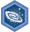## FANDOM

1,895 Pages

Algebra I: Introduction to algebraDescription
Achieve mastery in all skills in Algebra I: Introduction to algebra
Details
TypeEnergy point(s) awarded 0
Status Available
Disclosed?
Repeating?

This page contains exercises which pertain to the subcategory Algebra I: Introduction to algebra. Mastering them awards user with the Challenge Patch Algebra I: Introduction to algebra as well.

All items (13)

C
•Combining like terms with distribution and negative numbers
•Combining like terms with negative coefficients
D
•Defining appropriate units
•Dependent and independent variables
E
•Equivalent expressions with distribution and combining like terms
•Evaluating expressions in one variable
•Evaluating expressions with two variables
•Evaluating expressions with variables word problems
I
•Interpreting linear expressions
M
•Manipulating basic expressions with rational coefficients
N
•Nested fractions
W
•Writing expressions with variables 2
•Writing expressions with variables and parentheses